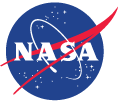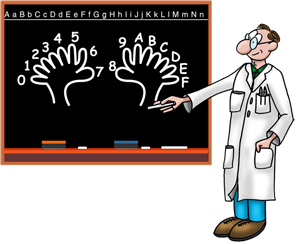# Why is sixteen so sweet?

Why is sixteen so sweet?Long ago, humans developed a counting system based on 10's for a very good reason. We have 10 fingers! We always have a "handy" way to keep track of things.

But this system doesn't work so well for computers. So another system was invented: hexadecimal, or "hex."

"Hexadeci-" means 16. Instead of only 10 digits (0 to 9), hex has 16 digits (0 to 15). Hex uses letters for the digits over 9:

A = 10
B = 11
C = 12
D = 13
E = 14
F = 15

Instead of converting the spacecraft's data from binary to decimal numbers, engineers convert from binary to hex.

Now why in the world would they do that?

Well, not every piece of information that goes into or comes out of a computer is really a number. Sometimes it really IS true or false, on or off, or one of a "multiple choice." Sometimes the information is a word. It turns out that hex and binary were made for each other.

So we can more easily talk, here are a few terms. A single binary digit is called a bit. Four bits grouped together are called a nybble. And two nybbles make (what else?) a byte!

If you arrange the bits in a nybble every possible way, you get only 16 possibilities.

## These are the hex numbers 0 through 15:

0000 = 0
0001 = 1
0010 = 2
0011 = 3
0100 = 4
0101 = 5
0110 = 6
0111 = 7
1000 = 8
1001 = 9
1010 = 10 (or A in hexadecimal)
1011 = 11 (B)
1100 = 12 (C)
1101 = 13 (D)
1110 = 14 (E)
1111 = 15 (F)

See? We know that the hex number 9 can only be 1001 in binary. And the hex number A can only be 1010.

A single binary digit is called a bit. Four bits grouped together are called a nybble. And two nybbles make (what else?) a byte!

Hex turns out to be a great way to compress data. You can put four separate pieces of information in just one hex digit.

So a spacecraft engineer might say that, in a particular nybble, the first bit means a heater switch is set to ON (if 1) or OFF (if 0). The second bit might mean a dust particle did (1) or did not (0) hit a tiny sensor. And so on.

Decimal numbers just aren't as handy. For example, look at these:

 Hex Binary Decimal 9999 1001 1001 1001 1001 39,321 AOAO 1010 0000 1010 0000 41,120 AAAA 1010 1010 1010 1010 43,690

What does the number 39,321 tell you about the binary number that is its equal? Not much without a special calculator to translate! But the hex number 9999 tells you exactly which bits are ones and which bits are zeroes!

So, you see, math systems are really like languages. We invent them to serve our purposes. And it is upon the language of hexadecimal numbers that almost all computers—and spacecraft—are now based!

information last updated more than 12 years ago